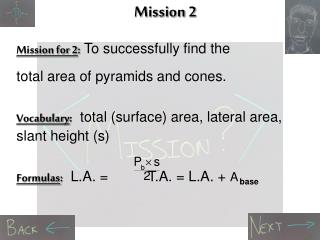Download PresentationMission 2

# Mission 2 - PowerPoint PPT PresentationDownload Presentation## Mission 2

- - - - - - - - - - - - - - - - - - - - - - - - - - - E N D - - - - - - - - - - - - - - - - - - - - - - - - - - -
##### Presentation Transcript

1. Mission 2 Mission for 2: To successfully find the total area of pyramids and cones. Vocabulary: total (surface) area, lateral area, slant height (s) Formulas: L.A. = T.A. = L.A. + Abase

2. Formula for L.A. The formula for the lateral area (L.A.) of a regular pyramid or cone is the half product of its slant height s and the perimeter p of its base. L.A. =

3. Formula for S.A. The formula for the surface area (S.A.) of a regular pyramid or cone is the sum of its lateral area and the area of its base (A). T.A.= L.A.+ Abase

4. Let’s Try one Find the surface area of a cone with a radius of 10 and a slant height of 13. Click on “Freckle Boy” after you’ve finished the problem.

5. Here is your Answer... T.A. = L.A. + Abase = +  r2 = +  r2 = + (10)2 = 408.41 + 314.16 = 722.57 units2

6. Here is another one! Find the lateral area of a square pyramid with a slant height of 14 and a base edge of 25.

7. The answer you have selected is incorrect. The correct answer was 700. Don’t worry. . . The little guy has the answer!